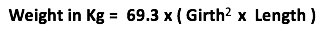# Calculate the “Live” Weight of your Pig

To calculate the live weight of a pig you need to make two measurements, Length and Girth then use this formula to calculate the weight.If calculating manually, use meters are the measure and not cm. i.e 1.2m and not 120cm

You need to measure the length and girth as shown in the picture below

To save you doing the math, if you use the sliders below to input the measurements, it will automatically calculate the weight for you. Happy Measuring 🙂